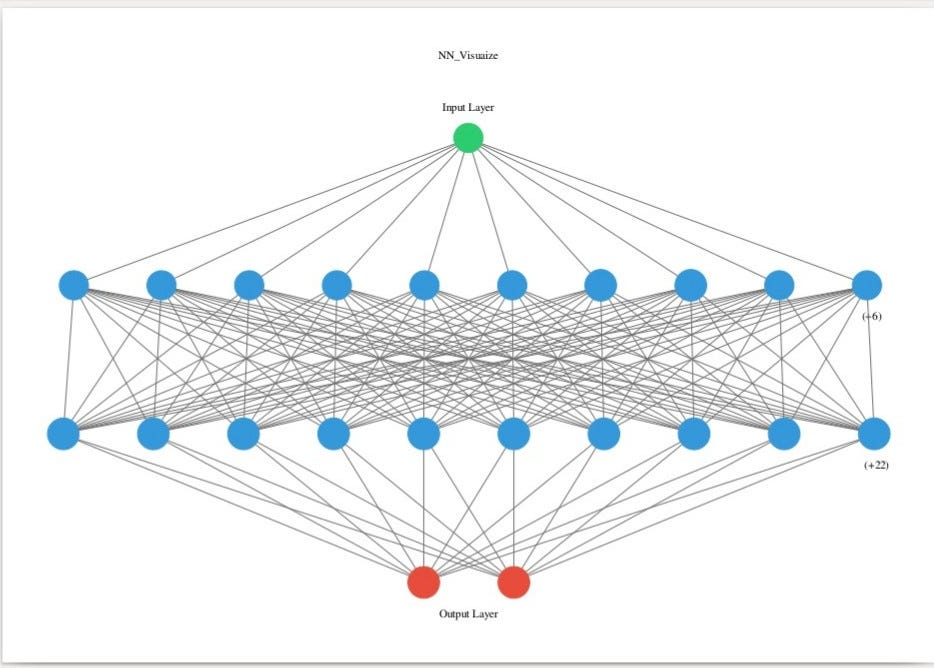# Implementing a Simple Neural Network using Keras

Hey there! so today i m goin to show how easy it is to implement a basic neural network in keras using python on my self made data for classifying the numbers as even or odd. So before starting i will do mark my steps and also explain u the code which i wrote.

So i hope that the readers do under stand the neural network concept and if not you must read this blog first https://towardsdatascience.com/the-mostly-complete-chart-of-neural-networks-explained-3fb6f2367464 .
So in neural network it is again as important to transform your data in such a way that or prepare your data like in accessible file format then correcting its missing data and fixing the anomilies and finally preprocessing / one hot encoding the data to make it all b/w 0–1 which we do to implement machine learning algos.

The steps which has to followed to implement your model prefectly should be in the following way :-
1.State the question or your final motive that you want to implement with your data and determine the required data.
2. Acquire data in accessible file format.
3. Identify and correct missing data points and fix the anomalies.
4. Prepare data for the model(one hot encoding)
5. Establish a baseline model that you want to establish.
6. Train your model on training data.
7. Make predictions using test data.
8. Calculate the preformance metrics.
9. If the performance is not satisfactory adjust the model like acquire more data or try different modeling technique.
10. Interpret the model visually and numerically and get the confusion matrix for final o/p.

So thats all the pre-requisites now coming to the most happiest part implementing this using python script by steps.
1.)import numpy as np
from sklearn.preprocessing import MinMaxScaler
here the minmaxscaler is used to preprocess the data in a range of 0–1 so that while our nodes in hidden layers do’nt face problems in calculations of big numbers.
2.)train_labels = [1, 2, 3, 4, 5, 11, 12, 13, 14, 15]
test_labels = [1,0,1,0,1,1,0,1,0,1]
here i gave a train set and the labels , 1 for odd and 0 for even .
3.)train_labels = np.array(train_labels)
test_lables = np.array(test_labels)
convert ur list into array.
4.)scaler = MinMaxScaler(feature_range=(0,1))
scaled_train = scaler.fit_transform((train_labels).reshape(-1,1))
Preprocessing the train_labels b/w 0–1.
5.)Now till here we are done making our data in its perfect format.Now we will implement neural network on this. So import following:-

import
keras from keras
import backend as
from keras.models import Sequential
from keras.layers import Activation
from keras.layers.core import Dense
from keras.metrics import categorical_crossentropy

as we implement our code you ll understant every modules that i have imported.
6.)model = Sequential([Dense(16,input_shape=(1,),activation=’relu’),
Dense(32,activation=’relu’),Dense(2,activation=’softmax’)])
model.summary()
*here we create our skeleton of model by inserting the layers, no of neurons in each layer and activation functions.
*Sequential() here is a construtor which allows us to insert layers.